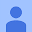# ALGEBRATOR - camera math solver that shows steps

In-app purchases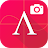3.2
3.46K reviews
1M+Everyone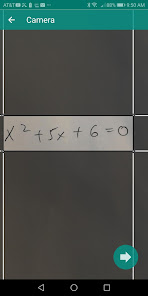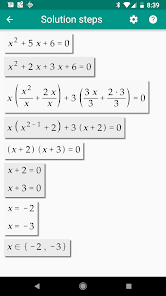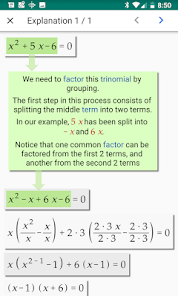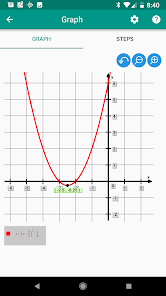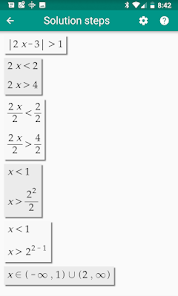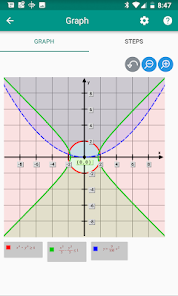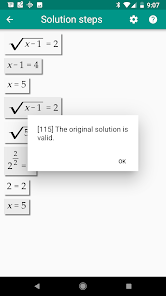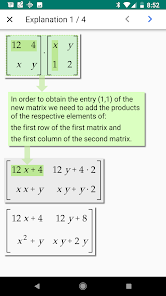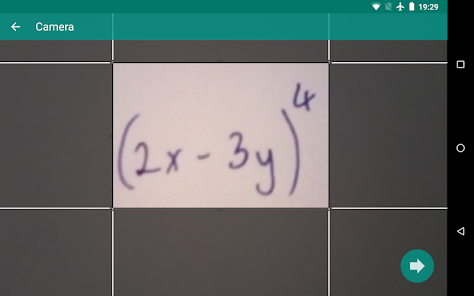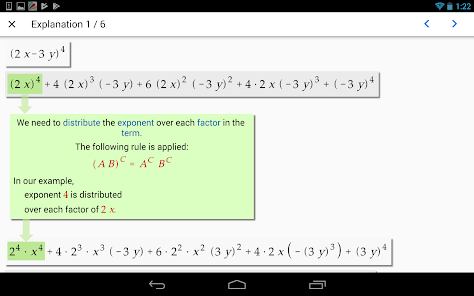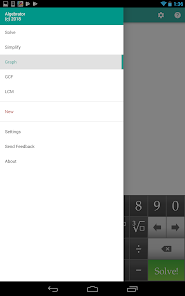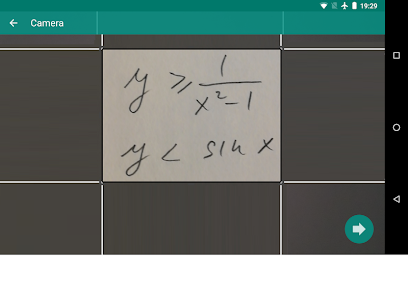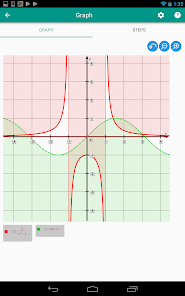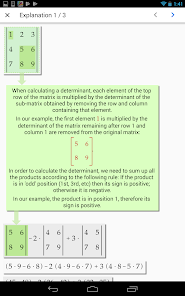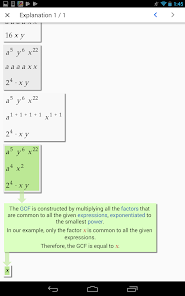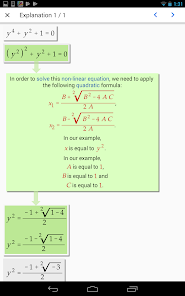Take a photo of your math problem and Algebrator does the rest!
We have been helping high-school and college students conquer math for more than e decades. Our camera algebra calculator shows you all steps of the solution as well as explanations to any math textbook problem you enter. This camera calculator app solves math homework problems just like your teacher! Explanations will tell you not only which algebra rule is being used, but also how it is applied in your particular math problem. Snap the problem picture or enter it via user friendly math keyboard to get instant steps and solution!

Fraction calculator will show steps to add, subtract and multiply fractions, as well as complex fraction division. After reducing fractions or rational numbers to simplest terms, fraction to decimal calculator will assist you with converting fractions to percentages. Lowest common denominator (lcm, lcd) calculator will help make equivalent fractions. Simplifying fractions also includes rationalizing denominators and numerators.
Graphing calculator will graph lines, horizontal and vertical asymptotes, x and y intercepts. It will produce graphs of quadratic equations, functions and parabola equations, linear equations (lines) and inequalities, trig. functions (e.g. cos graph) and curves such as hyperbola and circle equations. The graphing calculator math app will also show fractions on the number line, find the slope of a line as well as a parabola vertex. After placing polynomials in standard form, it will graph any polynomial function. Many other functions such as logarithmic, exponential and absolute value can also be graphed.
Equations and inequalities can be solved by several math equation solvers (e.g. quadratic equation calculator, linear equations calculator and system of equations solver (solving by substitution and addition)). Quadratic calculator will put a quadratic equation in standard form and then either use quadratic formula or solve by factoring. Logarithmic, rational, exponential, linear and quadratic equation solver will solve for x or any other math variable. Systems of linear equations and inequalities will be graphed to produce visually appealing math solution. Linear inequalities, polynomial equations, absolute value equations and inequalities are all solved by our algebra solver that shows work just like your teacher.
Factoring solver can factor polynomials, trinomials and binomials. It will factor quadratic equation so that it can be solved by the equation solver. Many different factoring methods such as factoring by grouping, difference of two squares, difference of two cubes, sum of two cubes are used. Greatest Common Factor calculator and perfect square trinomials are also used in the polynomial factoring calculator.
Trigonometry solver simplifies algebraic expressions with trig functions (such as sin or cos). It knows special values on trig unit circle (e.g. cos 0, sin 0, cos 1, sin 1, cos 30, sin 30, cos 45, sin 45, cos 90, sin 90). Sin cos tan and other trig functions can be simplified and graphed.
Exponents calculator applies exponent power rules and laws of exponents. It multiplies and divides fractional and negative exponents, using the properties of exponents. It also knows logarithm rules to apply to logarithmic functions.
Simplify expression calculator can simplify any algebraic or math expression, showing you all the step in the process. This includes complex numbers and imaginary numbers that are handled by calculator for complex numbers.
Radical simplifier includes cube and square root solver.
Square root properties are used while solving radical equations and simplifying radical expressions.
Matrix solver can multiply matrices, find inverse matrix and perform other matrix operations. Matrix determinant calculator can find any determinant value.
If you need help with math then our free math app is the here for you. Softmath has provided help with Algebra 1, Algebra 2 and College Algebra since 1990.
Updated on
Jun 26, 2022

## Data safetyNo information available
3.2
3.46K reviews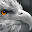Michael Navarro
June 7, 2021
The features are great and it's incredibly useful for studying. I'm always impressed by the scan function's accuracy. I couldn't access the paid version's features right away after upgrading but they responded very quickly once I made my situation heard and I'm happy with my purchase.
22 people found this review helpful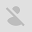05qjoan
February 21, 2021
This app converts your mobile phone into a great calculator, you have the usual options in a scientific calculator, but its integration allows you to do complex math, as well as if you change the value, it will do all the calculations again, useful if you need to use the same formula more than once. It does add, but they're usually located at the bottom of the screen.
44 people found this review helpful